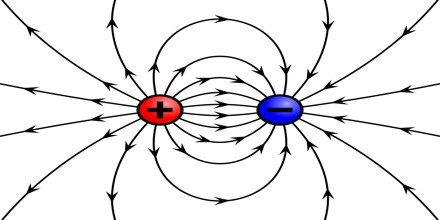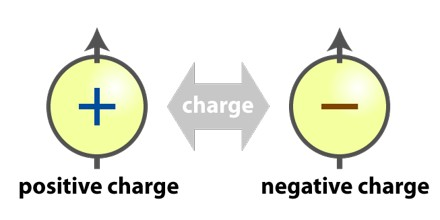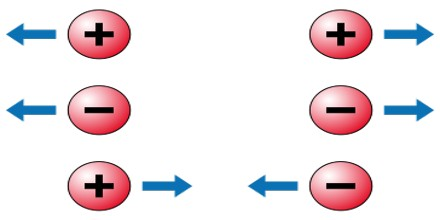Electric Charge

Definition

Electric charge is basic property of matter carried by some elementary particles. Electric charge, which can be positive or negative, occurs in discrete natural units and is neither created nor destroyed. Most electric charge is carried by the electrons and protons within an atom. Electrons are said to carry negative charge, while protons are said to carry positive charge, although these labels are completely arbitrary. Protons and electrons create electric fields, which exert a force called the Coulomb force, which radiates outward in all directions.In physics, charge, also known as electric charge, electrical charge, or electrostatic charge and symbolized q, is a characteristic of a unit of matter that expresses the extent to which it has more or fewer electrons than protons. In atoms, the electron carries a negative elementary or unit charge; the proton carries a positive charge. The two types of charge are equal and opposite. The electric charge is a fundamental conserved property of some subatomic particles, which determines their electromagnetic interaction. Electrically charged matter is influenced by, and produces, electromagnetic fields. The interaction between a moving charge and an electromagnetic field is the source of the electromagnetic force, which is one of the four fundamental forces. The electric charge of a macroscopic object is the sum of the electric charges of the particles that make it up. This charge is often small, because matter is made of atoms, and atoms typically have equal numbers of protons and electrons, in which case their charges cancel out, yielding a net charge of zero, thus making the atom neutral.

Types of Electric Charge

Electric charges are of two general types: positive and negative.Positive Charge – Positive Charge is carried by the protons in material and negative charge by electrons. The overall charge of an object is usually due to changes in the number of electrons. To make an object:

• Positively charged: electrons are removed making the object electron deficient.
• Negatively charged: electrons are added giving the object an excess of electrons.

Negative Charge – If the same amounts of negative and positive charge are found in an object, there is no net charge and the object is electrically neutral. If there is more of one type of charge than the other on the object then the object is said to be electrically charged.

Sometimes macroscopic objects contain ions distributed throughout the material, rigidly bound in place, giving an overall net positive or negative charge to the object. Also, macroscopic objects made of conductive elements, can more or less easily, depending on the element to take on or give off electrons, and then maintain a net negative or positive charge indefinitely. When the net electric charge of an object is non-zero and motionless, the phenomenon is known as static electricity. The law of conservation of charge always applies, giving the object from which a negative charge is taken a positive charge of the same magnitude, and vice versa.

Units of Electric Charge

The unit for measuring electric charge is the coulomb (C), named after Charles-Augustin Coulomb, an 18th-century French physicist. Coulomb developed the law that says “like charges repel; unlike charges attract.” A coulomb is defined as the amount of charge transported by a current of one ampere for one second. While this sounds like a small amount, according to HyperPhysics, “Two charges of one coulomb each separated by a meter would repel each other with a force of about a million tons!” Electrical engineers often prefer to use a larger unit for charge, the ampere hour, which is equal to 3,600 C.

The Coulomb force is one of the two fundamental forces that is noticeable on a macroscopic scale, the other being gravity. However, the electrical force is much, much stronger than gravity. The repulsive Coulomb force between two protons due to their charge is 4.1 × 1042 times stronger than the attractive gravitational force between them due to their mass. This is true at any distance, since the distance cancels out on both sides of the equation.Other Properties of Electric Charge

Electric charge is quantized, meaning that it occurs in discrete units. Protons and electrons carry charges of ±1.602 × 10−19 C. Every accumulation of charge is an even multiple of this number, and fractional charges cannot exist. Quantum chromodynamics (QCD) states that protons and neutrons are each composed of three quarks with charges of +2/3 or −1/3 of the unit proton charge, and two of one and one of the other combine to form particles with charges of zero or +1 unit charge.

Electric charge is a conserved quantity. That means it cannot be created or destroyed, and the net amount of electric charge in the universe is constant and unchanging. Positive and negative charges can neutralize each other, or neutral particles can split to form positively and negatively charged pairs of particles, but the net amount of charge always remains the same.

Static electricity and electric current are two separate phenomena. They both involve electric charge, and may occur simultaneously in the same object. Static electricity refers to the electric charge of an object and the related electrostatic discharge when two objects are brought together that is not at equilibrium. An electrostatic discharge creates a change in the charge of each of the two objects. In contrast, electric current is the flow of electric charge through an object, which produces no net loss or gain of electric charge.